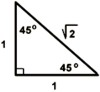PRECALC

Prof.  G. Battaly, Westchester Community College, NY

 Homework Problems PowerPoint lessons Class Notes Step-by-Step Trig Flash cards Links Schedule

 Step-by-step Instructions:
 PowerPoint Lessons These are read only.  If the file does not appear to open right away, please look for a small window to make the selection to read only. Class Notes 6.1 Basic Trig Identities 6.2 Double and Half Angles

Step by Step Instructions

Evaluate Functions 1.  Replace the independent variable, x, with place holders.
2.  Insert value or variable or function in the placeholder.
3.  Complete the algebraic evaluation.
eg:  G:  f(x) = 3x - 1   F:  f(2),  f(a+1)
f(   )  =  3 (      )  -1
f(2)    =  3(2) - 1   = 5
f(a+1) = 3(a+1) - 1 = 3a + 3 - 1 = 3a +2
Solving Rational Eq. 1.  Find LCD (factor if necessary)
2.  Is check required?  MUST check if LCD contains a variable.
3.  Use Multiplication Property of Equality to multiply all terms by the LCD.
4.  Simplify using distributive property or combining like terms, etc.
5.  Solve the equation.
6.  Check if MUST.  If checks correctly, then step 5 solution is the answer.
If does not check correctly, then there is no solution.
Sketching Polynomials1.  Determine degree n, and leading cooeficient, an of the polynomial
2.  Overview sketch:  a)  end behavior,  b) max # zeros,  c) max # extrema
3.  Find zeros.
4.  Set up table of intervals using the zeros as endpoints and selecting
convenient values of x within the intervals.
5.  Find the corresponding y values or each x selected above,
and enter the y value and the ordered pair (x,y) into the table.
6.  Plot the known points, including the zeros.
7.  Use your overview, together with the plotted points to complete an
estimated sketch.  Note that we do not know the exact locations of
extrema, only approximations.
Synthetic Division

P(x)
x – c

1.  Determine c.
2.  Determine degree, n.
3.  Rewrite P(x) in order of descending exponents.
4.  Are all exponents < n present?
If not, insert the missing terms with 0 as the coefficient.
5.  Set up the SD problem.
6.  Add an + 0 in row2 and place the sum below the line.
7.  Multiply the sum by c and place the product above the line in the next column.
8.  Add the next column and repeat steps 7 & 8 until you have a sum in the last column.
10.  The sum in the last column is the Remainder, R(x)
11.  The degree of the resulting quotient is n-1.
12.  Write the quotient using the bottom row as coefficients.

Exact Values of Trig Functions
of Special Angles30°=π / 6       60°=π / 345°=π / 4
1.  Sketch the x-y coordinate system.
2.  Draw the given angle.
a)  Use the quadrantal angles as reference:
π / 2 = 90°     π = 180°     3π / 2 = 270°      2π =  360°
b)  Determine the location of the terminal ray.
3.  Drop a perpendicular to form the right triange.
4.  Label the sides and hypotenuse.
a)  For the magnitude, use measures associated with the special triangle.
in degrees:         sin 30° = 1/2             tan 45° = 1
in radians:          sin π/6  = 1/2            tan π/4 = 1

b)  For each side use the sign appropriate for the quadrant.